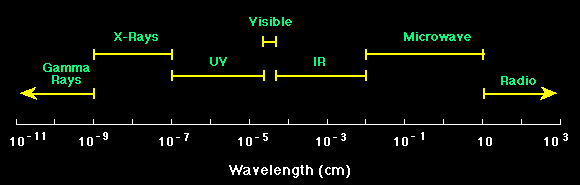Electromagnetic spectrum

The varieties of electromagnetic radiation form a continuum known as the Electromagnetic Spectrum. Its broad categories are called

• microwaves
• infrared light
• visible light
• ultraviolet light
• X-rays
• gamma rays
The only difference between these manifestions of electromagnetic radiation is due to differences in the frequency and wavelength of the oscillations. These two quantities are related by the formula
(velocity) = (frequency) x (wavelength), which in this case reads c = f
l.

The types of electromagnetic radiation are listed above in decreasing order of wavelength, and hence increasing order of frequency. (When we study a little quantum mechanics later in the course, we will learn that this is also the order of increasing energy for the individual photons.)